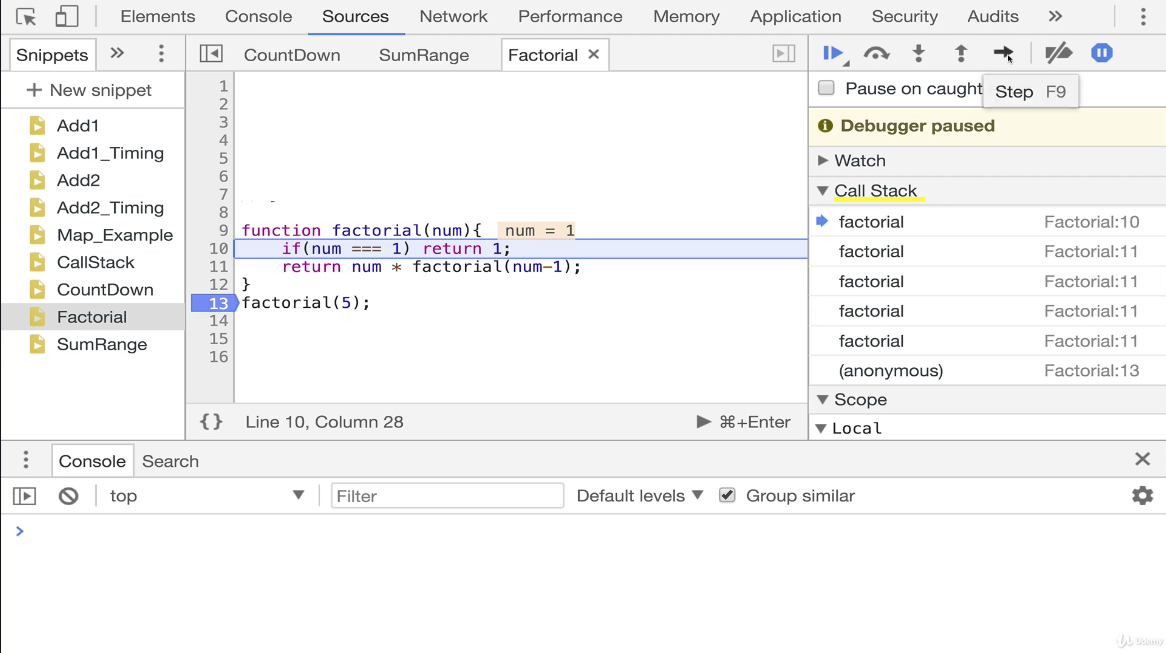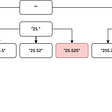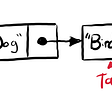# Recursion

## Overview & Example

What is Recursion?

A recursive function is a function that calls itself. It’s important to learn recursion because it’s used in common built-in JavaScript methods such as JSON.parse, JSON.stringify, and document.getElementById (Steele). Also, recursion is essential to understanding Tree and Graph traversal algorithms, which we will discuss in future blogs.

What is the Call Stack?

Before diving any deeper into our discussion of recursion, it’s helpful to discuss the call stack. A call stack is used in most programming languages. It is a, “… built in data structure that manages what happens when functions are invoked” (Steele).

When functions are invoked, they are placed on the top of a call stack. To help visualize this, think of stacking boxes. In order to get to the first box, you need to take off the boxes stacked on top of it.

How this relates to recursion is that “When we write recursive functions, we keep pushing new functions onto the call stack!” (Steele). Or, more precisely, we are pushing the same function onto the call stack over and over again. Now, you may be thinking this sounds like it would produce an infinite loop. However, as we will see in the next section, there are two key parts of a recursive function, one of which prevents an infinite loop from happening and another that pushes functions onto the call stack.

Essential Parts of a Recursive Function

The two essential parts of a recursive function are the recursive call and the base case. The recursive call is in the body of our function and where the function is calling itself and pushing the function onto the call stack. The base case is also in the body of our function and is the stopping point for our recursive calls (Learn). If there were no base case, the function would be in an infinite loop and run forever. Moreover, this is referred to as a stack overflow because too many functions are being put on the call stack (Steele).

Recursion in Code

Now that we know the essential parts of a recursive function and its impact on the call stack let’s see it in code.

To demonstrate recursion, we will write a function that mimics a factorial. For those who may have forgotten their 7th grade math class, a factorial is, “…the product of all positive integers less than or equal to n” (Wikipedia). The factorial of 5 for example is 5! = 5 x 4 x 3 x 2 *1 = 120.

`function factorial(num) {// This line represents the base case. We want our recursive function to stop when num = 1 because if we multiply by 0 our output becomes 0 and factorials only include positive integers. Also, if we don't stop our recursive function we will have a stack overflow/infinite loop as we continue past 1 and onto 0 and negative numbers.     if (num === 1) return 1;// This line represents our recursive call and num is decrementing by 1 each time until the base case condition is met, 1 in our case. See image below to see how the recursive calls are stacked on top of each other in the call stack.   return num * factorial(num -1)}factorial(5)Output: 120`Call Stack Image provided by lecture material presented in Colt Steele’s Udemy Course

The image above uses Google Chrome’s dev tools and demonstrates how our recursive function stacks our factorial function in the call stack and does not begin evaluating until we reach our base case. In the above example, the first item off of our call stack is the base case return 1. The next action to take place is multiple 1 by 2, then multiply 2 by 3, then 6 by 4, and lastly multiply 24 by 5 to reach out result of 120. The key thing to notice here is that multiplying by 5 is last to be evaluated, but the first added to the call stack. Conversely, the base case is last to be added to the call stack but the first to be evaluated. Remember back to the comparison between recursive functions and stacking boxes from earlier.

Thank you for taking the time to learn more about recursion. I hope you now understand the importance of recursion and apply it as you write and interpret code.

Resources

Steele, C. (n.d.). JavaScript Algorithms and Data Structures Masterclass. Online Course.

Recursion. (n.d.). Retrieved November 11, 2020, from https://learn.co/lessons/recursion-readme

Factorial. (2020, November 11). Retrieved November 11, 2020, from https://en.wikipedia.org/wiki/Factorial

--

--

--

## More from The Startup

Get smarter at building your thing. Follow to join The Startup’s +8 million monthly readers & +756K followers.

## Reclame — A Modular UI Component Architecture## 2 Modules in Nebula Graph: Scheduler & Executor## Live: JS Kongress meets Keerthana Krishnan & Felicitas Kugland## Top 10 Frequently made mistakes while working with Angular 2+## Image Crop — using React JS## Automatic API documentation in Node.js Using Swagger## Matthew Sedlacek

Software Engineer — Full Stack, JavaScript, ReactJS, Ruby on Rails, OO Programming (https://www.matthewsedlacek.com/)

## Selection Sort🛫## LeetCode 93 : Restore IP address — medium## Boruvka’s Algorithm